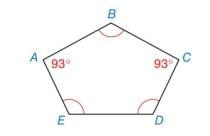Chapter 2.5, Problem 4E### Elementary Geometry for College St...

6th Edition
Daniel C. Alexander + 1 other
ISBN: 9781285195698

#### Solutions

Chapter
Section### Elementary Geometry for College St...

6th Edition
Daniel C. Alexander + 1 other
ISBN: 9781285195698
Textbook Problem
25 views

# In pentagon ABCDE with ∠ B ≅ ∠ D ≅ ∠ E , find the measure of interior angle D.To determine

The measure of interior angle D.

Explanation

Given:

A pentagon ABCDE with BDE.

Figure (1)

Formula used:

Write the equation to calculate the sum of the measures of the interior angles of a polygon.

S=(n2)×180°.

Here, S is the sum of the measures of interior angles of a polygon and n is sides of the polygon.

Definition:

If the angles of a pentagon are congruent, then the measures of the angles are same.

B=D=E.

Calculation:

Calculate the sum of the measures of interior angles of a polygon.

Substitute 5 for n in the above mention equation to find the sum of the measures of interior angles.

S=(52)×180°=3×180°=540°

From the figure, the sum of interior angles of pentagon is,

mA+mB+mC+mD+mE=540°.

Since, the angles of the pentagon are congruent then,

### Still sussing out bartleby?

Check out a sample textbook solution.

See a sample solution

#### The Solution to Your Study Problems

Bartleby provides explanations to thousands of textbook problems written by our experts, many with advanced degrees!

Get Started

#### Find more solutions based on key concepts